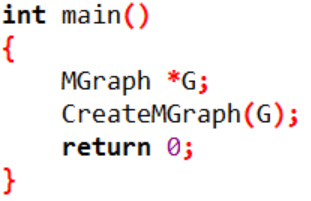#define MaxVertexNum 20 /* 最大顶点数设为20 /
#define INFINITY 32767 /
∞设为双字节无符号整数的最大值32767*/
typedef char VertexType; /* 顶点类型设为字符型 /
typedef int EdgeType; /

enum GraphType { DG, UG, DN, UN };

/

typedef struct {
VertexType Vertices[ MaxVertexNum ]; /* 顶点表 /
EdgeType Edges[ MaxVertexNum ］[ MaxVertexNum ]; /

int n, e; /

enum GraphType GType; /

} MGraph; /
MGragh是以邻接矩阵存储的图类型 /
void CreateMGraph ( MGraph *G )
{

int i, j, k, w;
G-> GType = UN; /
Undirected Network 无向网图 /
printf( "请输入顶点数和边数(输入格式为:顶点数, 边数):\n" );
scanf( "%d, %d",&(G->n), &(G->e) ); /

printf("请输入顶点信息(输入格式为:顶点号):\n");
for ( i = 0; i < G->n; i++ )
scanf( "%c",&(G-> Vertices[i]) ); /

for ( i = 0; i < G->n; i++ )
for ( j = 0; j < G->n; j++ )

G->Edges[i][j] = INFINITY; /

printf( "请输入每条边对应的两个顶点的序号和权值，输入格式为:i, j, w:\n" );
for ( k = 0; k < G->e; k++ ) {
scanf("%d,%d,%d ",&i, &j, &w); /

G->Edges[i][j] = w;
G->Edges[j][i] = w; /

}
}1个回答``` #include<stdio.h> #include<malloc.h> #define max 10000 int edge={0}; typedef struct _enode { //int dis; int vex; struct _enode* nextedge; }enode; typedef struct _vnode { int vex; enode* firstedge; }vnode; typedef struct _pic { int vex_num; int edge_num; vnode node[max]; }pic; void find_insert(enode* edge,enode* temp) { enode* p; p=edge; while(p->nextedge!=NULL) p=p->nextedge; p->nextedge=temp; } pic* create_pic() { int i=0,c1,c2; enode* node_temp; pic* picture; picture=(pic*)malloc(sizeof(pic)); picture->edge_num=5; picture->vex_num=3; for(i=1;i<=picture->vex_num;i++) //you are sb。I just say to you { picture->node[i].vex=i; picture->node[i].firstedge=NULL; c1=edge[i]; c2=edge[i]; node_temp=(enode*)malloc(sizeof(struct _enode)); node_temp->vex=c2; if(picture->node[c1].firstedge==NULL) { picture->node[c1].firstedge=node_temp; } else { find_insert(picture->node[c1].firstedge,node_temp); } } return picture; } int main(void) { int i=0; pic* picture; for(i=1;i<=5;i++) { scanf("%d",&edge[i]); scanf("%d",&edge[i]); } picture=create_pic(); for(i=1;i<=picture->vex_num;i++) { enode* p; p=(enode*)malloc(sizeof(enode)); if(picture->node[i].firstedge==NULL) printf("error"); else { p=picture->node[i].firstedge; printf("%d ",picture->node[i].vex); while(p!=NULL) { printf("%d ",p->vex); p=p->nextedge; } } printf("\n"); } } ``` 一个邻接表的建立与输出的程序，求大神看看为啥输入5个边的信息没有反应 ![图片说明](https://img-ask.csdn.net/upload/201703/08/1488960146_731655.png)

【数据结构】用邻接表存储图的简单问题

C++不带权无向网的邻接表的最小生成树的实现所用算法

**图有n个顶点和e条边，建立邻接表的复杂度为什么为O(n+e)呢？通过查找确定顶点在图中的位置，时间复杂度为什么为O(n*e)？**

Description 给出一个有n个顶点的无向图，顶点编号从0到n-1。给出每一条边，输出该图的邻接矩阵和邻接表。 Input 输入的第一行是顶点数n和边数 e 。 1 ≤ n ≤ 300 ，1 ≤ e ≤ 1000 接下来是 e 行，每行2个整数 i , j ( 0 ≤ i, j < n ) ，表示顶点 i 和 j 之间有一条边。 Output 输出该图的邻接矩阵。邻接表按顶点编号每行从小到大，每列也是从小到大。 然后输出一个空行。 接着输出该图的邻接表。 为了使得答案唯一，邻接表每行的第一个数字是顶点编号，然后按照顶点的下标编号从小到大输出各邻接顶点。 Sample Input 6 7 0 1 1 5 0 4 2 5 1 4 2 3 3 5 Sample Output 0 1 0 0 1 0 1 0 0 0 1 1 0 0 0 1 0 1 0 0 1 0 0 1 1 1 0 0 0 0 0 1 1 1 0 0 0 1 4 1 0 4 5 2 3 5 3 2 5 4 0 1 5 1 2 3

``` #include<stdio.h> #include<stdlib.h> #define MaxVnum 50 typedef char VertexType; typedef struct ArcNode{ int adjvex; double weight; struct ArcNode *nextarc; }ArcNode; typedef struct{ //头结点 VertexType data; ArcNode *firstarc; }AdjList[MaxVnum]; typedef struct{ // 图的类型定义 int vexnum,arcnum; // 实际的顶点数、边数 AdjList vertices; }Graph; int visited[MaxVnum]; void AddNode(Graph &G,int m,int n,float w){//表中添加n结点到m结点的路径 ArcNode *p,*q,*s; p=(ArcNode*)malloc(sizeof(ArcNode)); q=(ArcNode*)malloc(sizeof(ArcNode)); s=(ArcNode*)malloc(sizeof(ArcNode)); p->adjvex=n; p->nextarc=NULL; p->weight=w; q=G.vertices[m].firstarc; if(q==NULL){ G.vertices[m].firstarc=p;//第一个节点为空直接添加 } else{ while(q->adjvex<p->adjvex){//不为空时，后移实现节点索引的递增排列 s=q; q=q->nextarc; if(q==NULL){ break; } } if(q==NULL){ s->nextarc=p; } else{ if(q==G.vertices[m].firstarc){ s=G.vertices[m].firstarc; G.vertices[m].firstarc=p; p->nextarc=s; } else{ s->nextarc=p; p->nextarc=q; } } } } void GreateGraph(Graph &G){ int i,a,b; float c; printf("输入顶点数和边数："); scanf("%d %d",&G.vexnum,&G.arcnum); for(i=0;i<G.vexnum;i++){ G.vertices[i].data=i; } printf("请输入邻接表：\n"); for(i=0;i<G.arcnum;i++){ scanf("%d%d%f",&a,&b,&c); AddNode(G,a,b,c); } } void DFS(Graph G,int i){ ArcNode *p; visited[i]=1; printf("%c",G.vertices[i].data); p=G.vertices[i].firstarc; while(p){ if(!visited[p->adjvex]){ DFS(G,p->adjvex); } p=p->nextarc; } } void DFSTraverse(Graph G){ int i; for(i=0;i<G.vexnum;i++){ if(!visited[i]){ DFS(G,i); } } } int main(void){ Graph G; int i,j; GreateGraph(G); printf("用邻接矩阵存储图的深度优先遍历：\n"); DFSTraverse(G); } ```

java任意输入给一个邻接表，根据邻接表绘制图形。要求不能遮盖节点，希望大神给出完整代码

Problem Description Lady CA has a tree with n points numbered 1,2,...,n, and each edge has its weight. The unique route connecting two points is called a chain, and the length of a chain equals the sum value of the weights of the edges passed. The point number m is called the root. Lady CA defines a special kind of chain called folded chain, the chain connecting the points numbered x,y(x≠y) is called a folded chain, if and only if the chain connecting the point numbered x and the root doesn't pass the point numbered y, and the chain connecting the point numbered y and the root doesn't pass the point numbered x. Lady CA wants to find the length of the kth longest folded chain. Notice that the chain connecting the points numbered x,y and the chain connecting the points numbered y,x are the same. Input The first line contains an integer T(1≤T≤3)——The number of the test cases. For each test case: The first line contains three integers n(2≤n≤50,000),m(1≤m≤n),k(1≤k≤n×(n−1)2). Between each two adjacent integers there is a white space separated. The second line to the nth line describes the n−1 edges in the graph. Each line contains three integers u,v(1≤u,v≤n,u≠v),w(1≤w≤10,000), which means there is an edge which has a weight w connecting the points numbered u,v. Between each two adjacent integers there is a white space separated. Output For each test case, the only line contains the only integer that is the length of the kth longest folded chain. If the kth longest folded chain doesn't exist, print NO. Sample Input 2 5 1 3 1 2 8 1 5 4 2 3 5 2 4 6 5 1 5 1 2 8 1 5 4 2 3 5 2 4 6 Sample Output 12 NO

“亚马逊丛林里的蝴蝶扇动几下翅膀就可能引起两周后美国德州的一次飓风……” 这句人人皆知的话最初用来描述非线性系统中微小参数的变化所引起的系统极大变化。 而在更长的时间尺度内，我们所生活的这个世界就是这样一个异常复杂的非线性系统…… 水泥、穹顶、透视——关于时间与技艺的蝴蝶效应 公元前3000年，古埃及人将尼罗河中挖出的泥浆与纳特龙盐湖中的矿物盐混合，再掺入煅烧石灰石制成的石灰，由此得来了人...

C++11：一些微小的变化（新的数据类型、template表达式内的空格、nullptr、std::nullptr_t）

HashMap底层实现原理，红黑树，B+树，B树的结构原理 Spring的AOP和IOC是什么？它们常见的使用场景有哪些？Spring事务，事务的属性，传播行为，数据库隔离级别 Spring和SpringMVC，MyBatis以及SpringBoot的注解分别有哪些？SpringMVC的工作原理，SpringBoot框架的优点，MyBatis框架的优点 SpringCould组件有哪些，他们...

【阿里P6面经】二本，curd两年，疯狂复习，拿下阿里offer

《经典算法案例》01-08：如何使用质数设计扫雷（Minesweeper）游戏

《Oracle Java SE编程自学与面试指南》最佳学习路线图（2020最新版）